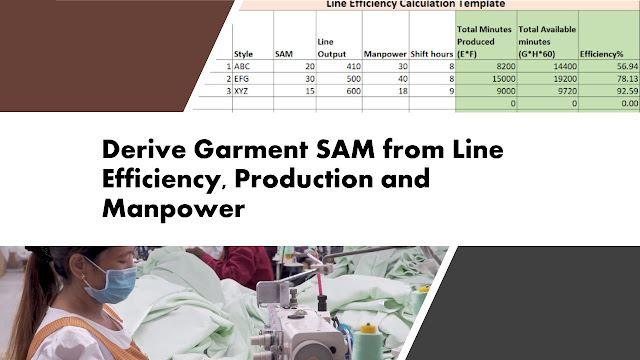# How to Derive Garment SAM from Production, Efficiency and Manpower Data?This question was posted on ASK OCS platform by an OCS reader.
I have these information - 32 operators, 8 helpers and 2 pressmen are working in a line. Line is working @45% efficiency and line output are 50 pcs/hr. How to derive garment SAM from this information?
First, let me clarify to you that garment SAM can't be calculated by the reverse method. Instead produced minutes and efficiency of a line are calculated using garment SAM. Sometimes, for the calculation purpose, you may need to derive SAM from efficiency data. In this article, I will show you how to derive garment SAM when you have information on manpower, production, and line efficiency.

We will use the line efficiency calculation formula for deriving garment SAM and following a reverse calculation method.

Efficiency %= (Production x SAM X 100)/(Manpower x Working hoursx60)
You have mentioned that you have line efficiency data (45%). I am assuming that the factory establishes garment SAM and they used SAM for calculating their line efficiency. But somehow you don't know the garment SAM factory is making currently. You only have the above information. You can derive SAM using the following steps.

Also, you need to be sure that helpers and pressman operations were assigned with SAM. In case helpers’ operations are not assigned with a SAM and not included in the Efficiency calculation, you should exclude them in manpower counting.

• Calculate total manpower (32+8+2 = 42)
• Total Hours Worked in an hour = 42 x 1 hours = 42x60 minutes
• Hourly production = 50 pieces
• Garment SAM (let's say) = Y
• SAM produced = 50xY
• Line efficiency = 45%

Therefore, as per the efficiency formula,

(Production x SAM X 100)/(Manpower x Working hours x 60)=Efficiency %
50Y*100/(42x60)=45
Y= 45x42x60/50*100=22.68 Minutes

SAM of this garment is 22.68 minutes. In the calculation, production is taken for one hour, so hours worked is also considered for 1 hour.  In case, you have the line output of 8 hours, you need to consider 8 hours per worker for calculating hours worked.  See more examples in the following table.

 Manpower Hours worked Line output Line Efficiency(%) Garment SAM 42 1 50 45 22.68 42 8 400 45 22.68 42 8 500 55 22.18 30 8 800 80 14.40 30 1 100 80 ? 35 8 1000 75 ?

I hope the calculation method is clear to you. If you have more questions, you can send your queries through ASK OCS.

Related Post: How to Calculate Hourly Production Target